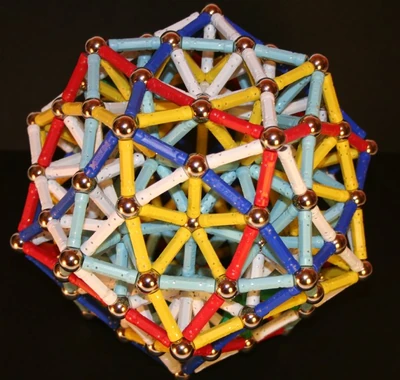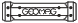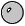## FANDOM

152 Pages

 Dodecahedron composed of 5 wobbly cubesExterior coloured to expose the cubes. Type Category:Dodecahedron Rods 432 ×Spheres 112 ×Author --kloniwotski 9, Dec., 2007

That the diagonals of the twelve pentagonal faces of a dodecahedron are also the edges of five cubes has long been my favourite geometric fact. This geomag model attempts to illustrate it, though the "diagonals" are somewhat curved and the "cubes", thereby, a shade on the wobbly side.

The construction starts with a regular pentagon. Either use a panel, or use a pentagonal pyramid to regularise it.

Add points on each of the sides, using ten more rods in total, making a five-pointed star shape.

Use another ten rods to make five triangles pointing up, one from each edge of the pentagon.

Use ten more rods to connect each of the points of the star to the upper point of the extra triangles, and then to a single ball slightly above the center of the pentagon (this will be the apex of the pentagonal pyramid, if you used that, or just a ball where that apex would have been if you used the panel.)

That makes one of the twelve stars needed for the construction. Proceed identically for the other eleven, except that where the points of the stars join, the rods connecting the star points to the upper point of the extra triangles are shared between all three stars joined at each vertex of the dodecahedron.

This may help to clarify the construction:

The inner surface of the supporting framework turns out to be a distortion of a rhombic triacontahedron, with the valency-5 vertices above the center of one of the pentagons in the outer surface.